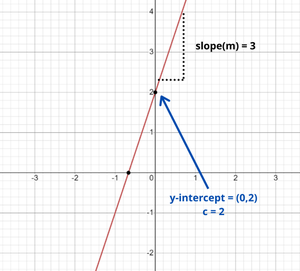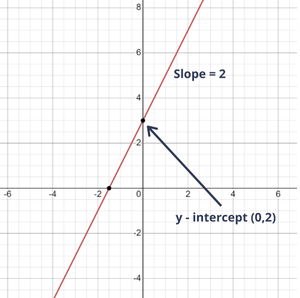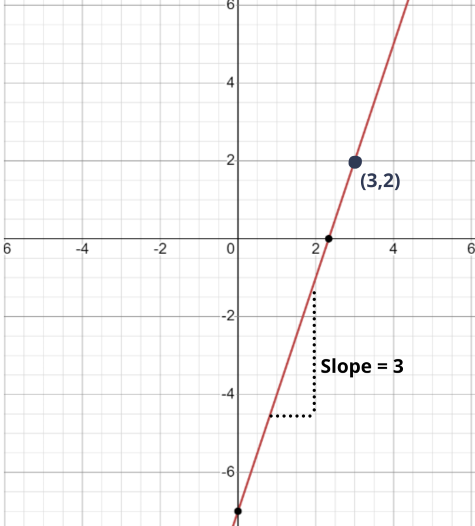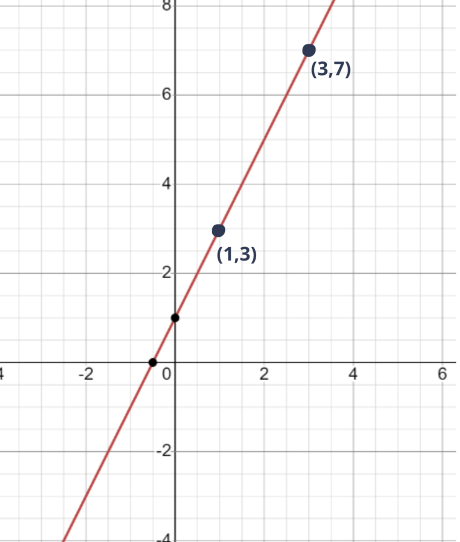# Writing Slope-Intercept Equations

• Last Updated : 30 Apr, 2021

Straight-line equations, also known as “linear” equations, have simple variable expressions with no exponents and graph as straight lines. A straight-line equation is one that has only two variables: x and y, rather than variables like y2 or √x. Because it contains information about these two properties, one type of straight-line equation is called the slope-intercept form.

The equation of a straight line is given as:

Attention reader! All those who say programming isn't for kids, just haven't met the right mentors yet. Join the  Demo Class for First Step to Coding Coursespecifically designed for students of class 8 to 12.

The students will get to learn more about the world of programming in these free classes which will definitely help them in making a wise career choice in the future.

y = mx + c

where m and c are constants, has a graph that is a straight line.

e.g.:

y = 3x / 2 + 5, y = 2 x + 7 etc.

### Slope-intercept form of a straight line

The slope-intercept form of a straight line is probably the most frequently used way to express the equation of a line. Slope-intercept form i.e. y = mx + c is the equation of a straight line whose slope is m and which makes an intercept c on the y-axis. Here m and c can be any two real numbers.

The slope (or gradient) of the line is defined by the value of m in the equation. It may be either a positive, negative, or zero value.

• Positive gradient lines slope upwards from left to right.
• Negative gradient lines slant backward from left to right.
• Horizontal lines have a zero gradient.

The value of c is called the vertical intercept of the line. It is the value of y when x = 0. When drawing a line, c gives the position where the line cuts the vertical axis.

e.g.: y = 3x + 2, here the slope of the line is 3 (i.e. m = 3) and it makes an intercept of 2 on the y – axis (i.e. c = 2).

In order to use the slope-intercept equation, all needed to do is that first find the slope of a line and secondly determine the y-intercept of a line.

Now, Let’s learn how to write the slope-intercept equation of a line, there are three different of it that are:

### 1.  Slope-intercept equation from a graph

Equations in slope intercept form are formed by combining the slope and y-intercept of a line to form the following equation: y = m x + c.

To build a slope-intercept equation, one will need to use a graph to locate both the slope (m) and the y -intercept (b). Let’s take a look at the process with the help of examples as:

Example 1: Find the slope-intercept form of line 3x – y + 2 = 0 from the graph shown below:Graphical representation of equation: 3x-y+2 = 0

Solution:

In the above figure, the slope of the line is 3 which makes an intercept of 2 on y-axis.

Now, if the equation of a line isn’t given, but the graph is given, then mark the x and y intercepts on the graph. Therefore, in the above graph

x-intercept is -2/3 and y-intercept is 2.

The slope of the line defined as the ratio of the change in y to the change in x between any two points on the line i.e.,

Slope = Change in y / Change in x

= (0 – 2) / (-2/3 – 0)

= 3

Therefore, the slope of the line is 3 which is making an intercept of 2 on y-axis. So, m is 3 and c is 2 and the equation of line from the graph is:

y = 3x + 2.

Example 2: Find the equation of the line having slope equal to 2 whose graph is shown below:Solution:

From the given graph,

The slope(m) of the line is 2, and it is having y-intercept at (0,3).

So, the value of c = 3.

Putting the values of m and c, in the equation of line as:

y = mx +c

= (2)x + (3)

= 2x + 3

Hence, the equation of the line is: y = 2x + 3.

### 2. Slope-intercept equation from slope and a point

If the slope of the line is given which is passing through a point (x1, y1), then equation of line can be given as:

y – y1 = m(x – x1)

This form of the equation of a straight line is also known as “point-slope” form.

Example 1: Find the equation of a straight line passing through the point (3,2) having slope equal to 3.

Solution:

The graph for the straight line passing through the point (3,2) having slope equal to 3 is:Given that,

Slope (m) = 3, and (x1, y1) = (3, 2)

The point-slope form is:

y – y1 = m(x – x1)

Now putting the values in the equation:

y – 2 = 3(x – 3)

= 3x – 9

y = 3x – 9 + 2

= 3x – 7

Hence, the equation of the line is: y = 3x – 7.

How can point-slope form be written as slope-intercept form?

Since, the slope-intercept form (i.e. y = mx + c) and point-slope form both are same equation but in different form.

The value of c (called the y-intercept) is where the line crosses the y-axis.

So point (x1, y1) is actually at (0, c) and the equation becomes:

y − y1 = m(x − x1)

(x1, y1) is actually (0, c): y − c = m(x − 0)

y − c = mx

y = mx + c

So, if one point on the line and the slope of the line is known, then the slope-intercept form as point-slope form can also be written.

Example 2: A line having slope, 4 and making its x-intercept at (5,0). Find its equation.

Solution:

Given that,

The slope (m) = 4, and (x1, y1) = (5, 0)

The point-slope form is:

y – y1 = m(x – x1)

Now putting the values in the equation:

y – 0 = 4(x – 5)

= 4x – 5

y = 4x – 5

Hence, the equation of the line is: y = 4x – 5.

### 3. Slope-intercept equation from two points

Example 1: Let’s consider a line passing through points (1, 3) and (3,7) as shown in the graph.Determine the slope-intercept form of the line.

Solution:

According to two-point form of a straight line, slope of the line can be given as:

m = (y2 – y1) / (x2 – x1

Substitute the given values in the above expression:

m = (7 – 3) / (3 – 1)

= 4/2

= 2

Now, substitute the value of m in slope-intercept form:

y = 2x + c

Now, y = 2x + c line passes through the points (1,3) and (3,7).

Substitute one of the coordinates in the equation:

y = 2x + c

3 = 2(1) + c

3 – 2 = c

c = 1

Thus, the equation of line is y = 2x + 1.

Example 2: Write the equation of the line passing through (4,7) and (6,13).

Solution:

Use the formula to find the slope between the two points.

Slope, (m) = (13 – 7) / (6 – 4)

= 6/2

= 3

Substitute 3 for m in the equation y = mx + c.

⇒ y = 3x + c

Substitute one of the coordinates in the above equation:

7 = 3(4) + c

c = -12 + 7

= -5

​Therefore, the slope-intercept form is given as: y = 3x – 5

### 4. Constructing linear equations from the context

Now let’s learn how to construct linear equations from the word problems.

Example 1: The local water supply has a leak, it is losing 4 gallons of water every second. After 15 seconds, the water level is at 1024 gallons. Write an equation representing this situation.

Solution:

The above problem can be represented in slope-intercept form i.e. y = mx + c, where the y-axis denotes the level of water and x-axis denotes the time in seconds.

Now, the water level is changing at 4 gallons per sec.

And since,

Slope = Change in y / Change in x

Therefore,

Slope = 4 gallons / 1 sec

= 4

But here water supply has a leak, and it is loosing 4 gallons per second, which means slope is decreasing, therefore slope of the line will be ‘-4’

Now, After 15 seconds, the water level was found to be at 1024 gallons.

So, by putting these values in y = mx + c, we get:

1024 gallons = (-4 gallons/ secs) × (15 secs) + c

1024 = -60 + c

c = 1084 gallons.

Now, putting the values of m and c to get the generalized equation of the line:

y = (-4) x + 1084

y = -4x + 1084

Hence, the above problem can be represented in a linear equation as: y = -4x + 1084

Example 2: Ram was hiking up a mountain. She started her hike at an elevation of 800 meters and ascended at a constant rate. After 5 hours, she reached an elevation of 1300 meters.

Solution:

Let y represent Ram’s elevation (in m) after x hours. Construct a linear equation for the relationship between elevation and number of hours.

Ram started her hike at 800 m, that means (0, 800) was her starting point. Looking closely at this point, the y-intercept of the graph between elevation (y-axis) and a number of hours (x-axis).

So, the value of c will be 800. Now, he is ascending at a constant rate and reaches 1300 m after 5 hours, i.e. he is at (1200, 5).

Now, using the two-point formula to find the slope of the line:

m = y2 – y1 / x2 – x1

= (1300 – 800) / (5 – 0)

= 500 / 5

= 100

Substitute 100 for m and 800 for c in the equation of slope-intercept form:

y = 100x + 800.

### Conclusion

To find the slope-intercept form of the line, one is needed to find:

1. The slope of the line and
2. The y-intercept of the line
• Now, if a graph of a line whose slope is ‘1’ and y-intercept at (0,5) is given, simply substitute m = 1 and c = 5 into the slope-intercept form.

⇒ y = x + 5

• Suppose, it is required to find the equation of a line passing through (3, 2) and having slope = 3, use point-slope form [i.e. y – y1 = m(x – x1) ] to find the equation of the line. Substitute the given values: y – 2 = 3 (x – 3)

⇒ y = 3x – 7

• If a line is passing through two points, use two point form to find the equation of the line.

e.g.: if a line is passing through points (1,3) and (2,5) slope of the line can be given by:

⇒ m = (5-3) / (2-1)

= 2

Putting the value of m and one of the coordinate in y = mx + c we get:

y = mx + c

3 = (2)1 + c

c = 1

So, the equation of the line will be y = 2x + 1.

My Personal Notes arrow_drop_up